# Sort Array in JavaScript

##### How to sort the elements of an array in JavaScript?

In the previous posts, we learnt about adding elements to the Array. In this post, we shall understand how to sort JavaScript array in different order.

### Array Sort Methods

The `sort()` method is used to sort the elements of an array in order and returns the new array. Sorting is nothing but putting all the elements of a list in a certain order. The different type of sort orders are alphabetic, numeric, ascending and descending. The default sort order of the `sort()` method is alphabetic order for the words and acending order for the numbers. This method works finely for the strings and changes the original array.

In JavaScript, by using `sort()` method we can sort the elements of an array.

#### Sorting an array alphabetically

```<p>Click the button to sort an array.</p>
<button onclick="myFunction()">Click</button>
<p id="myId"></p>

<script>
var a = ["India", "Pakisthan", "Bangladesh", "China"];
document.getElementById("myId").innerHTML = a;

function myFunction() {
a.sort();
document.getElementById("myId").innerHTML = a;
}
</script>
```

In the above code snippet we have given `Id` as "`myId`"to the second `<p>` element in the HTML code. There is a function myFunction() in the`<script>`block which is connected to the onclick of the HTML button and array with value "["India", "Pakisthan", "Bangladesh", "China"]" to the variable `a`. We need to sort the elements of an array, for that we are using `sort()` method in the `<script>` block. Onclick of the button "Click" in the HTML code fires the function myFunction() in the `<script>` block, at the same time `sort()` method sorts the order of the elements in an array and return the new array as output.

OUTPUT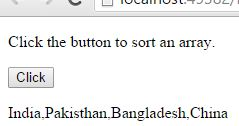The above output showing the array before sorting.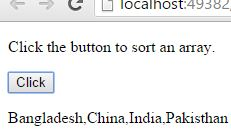The above output showing the array after sorting.

### Ascending order Sorting

Ascending order sorting means arranging the numbers from small to big, and it is by default in Array sort {`sort()` method}.

```<p>Click the button to sort an array in Ascending order.</p>
<button onclick="myFunction()">Click</button>
<p id="myId"></p>

<script>
var a = [85, 52, 56, 98, 74, 32, 5, 9, 99];
document.getElementById("myId").innerHTML = a;

function myFunction() {
a.sort(function (a, b) { return a - b }); //* Ascending order is default in sorting method, we can use directly a.sort() *//
document.getElementById("myId").innerHTML = a;
}
</script>```

Ascending order sorting

In the above code snippet we have given `Id` as "`myId`"to the second `<p>` element in the HTML code. There is a function myFunction() in the`<script>`block which is connected to the onclick of the HTML button and array with value "[85, 52, 56, 98, 74, 32, 5, 9, 99]" to the variable `a`. We need to sort the numbers of an array in ascending order, for that we are using `sort()` method like `.sort(function(a, b) {return a-b})` in the `<script>` block, insted of that we can use `a.sort()` directly because ascending order is default in sorting method. Onclick of the button "Click" in the HTML code fires the function myFunction() in the `<script>` block, at the same time `.sort(function(a, b) {return a-b})` method sorts and sets the numbers of an array in ascending order and return the new array as output.

OUTPUTThe above output is showing the numbers before sorting.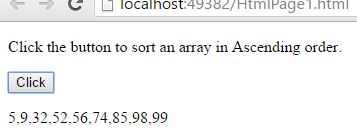The above output is showing the numbers afters sorting in ascending order.

### Descending order Sorting

Descending order sorting means arranging the numbers from big to small.

```<p>Click the button to sort an array in Descending Order.</p>
<button onclick="myFunction()">Click</button>
<p id="myId"></p>

<script>
var a = [85, 52, 56, 98, 74, 32, 5, 9, 99];
document.getElementById("myId").innerHTML = a;

function myFunction() {
a.sort(function (a, b) { return b - a });
document.getElementById("myId").innerHTML = a;
}
</script>
```

Descending order sorting

We need to sort the numbers of an array in descending order, for that we are using `sort()` method like `.sort(function(a, b) {return b-a})` in the `<script>` block. Onclick of the button "Click" in the HTML code fires the function myFunction() in the `<script>` block, at the same time `.sort(function(a, b) {return b-a})` method sorts and sets the numbers of an array in descending order and return the new array as output.

OUTPUTThe above output is showing the numbers before sorting.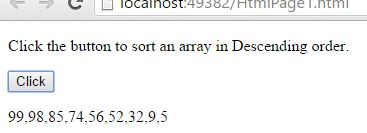The above output is showing the numbers after sorting in descending order.

### Highest value Sorting

Highest value sorting means arranging the numbers in order and returning the highest value from that numbers.

```<p>Click the button to sort an array for highest value.</p>
<button onclick="myFunction()">Click</button>
<p id="myId"></p>

<script>
var a = [85, 52, 56, 98, 74, 32, 5, 9, 99];
document.getElementById("myId").innerHTML = a;

function myFunction() {
a.sort(function (a, b) { return b - a });
document.getElementById("myId").innerHTML = a;
}
</script>
```

Highest value sorting

In the above code snippet we have given `Id` as "`myId`"to the second `<p>` element in the HTML code. There is a function myFunction() in the`<script>`block which is connected to the onclick of the HTML button and array with value "[85, 52, 56, 98, 74, 32, 5, 9, 99]" to the variable `a`We need to return the highest numbers of an array, for that we are using `sort()` method like `.sort(function(a, b) {return b-a})` in the `<script>` block, which sorts an array in descending order. Onclick of the button "Click" in the HTML code fires the function myFunction() in the `<script>` block, at the same time `.sort(function(a, b) {return b-a})` method sorts and sets the numbers of an array in descending order, from that descending order (Higher to Lower) `a` returns the first number (highest value) as output.

OUTPUT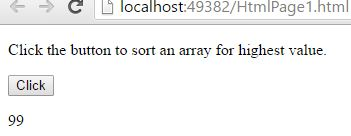### Lowest value sorting

Lowest value sorting means arranging the numbers in order and returning the small value from that numbers.

```<p>Click the button to sort an array in for lowest value.</p>
<button onclick="myFunction()">Click</button>
<p id="myId"></p>

<script>
var a = [85, 52, 56, 98, 74, 32, 5, 9, 99];
document.getElementById("myId").innerHTML = a;

function myFunction() {
a.sort(function (a, b) { return a - b });
document.getElementById("myId").innerHTML = a;
}
</script>
```

Lowest value sorting

We need to return the lowest numbers of an array, for that we are using `sort()` method like `.sort(function(a, b) {return b-a})` in the `<script>` block, which sorts an array in descending order. Onclick of the button "Click" in the HTML code fires the function myFunction() in the `<script>` block, at the same time `.sort(function(a, b) {return a-b})` method sorts and sets the numbers of an array in ascending order, from that ascending order (Lower to Higher) `a` returns the first number (lowest value) as output.

OUTPUT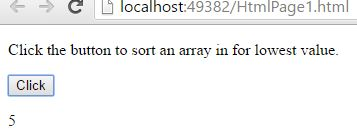### Reversing the sorted order

For reversing the sort order, first we need to sort the order and then reverse the sorted order.

```<p>Click the button to reverse the sort.</p>
<button onclick="myFunction()">Click</button>
<p id="myId"></p>

<script>
var a = ["India", "Pakisthan", "Bangladesh", "China"];
document.getElementById("myId").innerHTML = a;

function myFunction() {
a.sort();  //* Sort an array *//
a.reverse(); //* Revsre the sorted array *//
document.getElementById("myId").innerHTML = a;
}
</script>
```

Reversing the sort order

We need to reverse the sorted array, for that first we are sorting an array by using `a.sort()` method, after that reversing the sorted array by using `a.reverse()` in the `<script>` block. Onclick of the button "Click" in the HTML code fires the function myFunction() in the `<script>` block, at the same time `sort()` method sort an array after that `revrse()` method reversed the sorted array and returns as output.

OUTPUTThe above output is showing an array before sorting.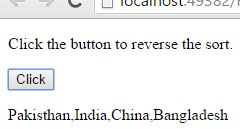The above output is showing the reversed sorted array.

Views: 8888 | Post Order: 156